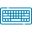## Table of Content

Feedback

Our radius of convergence calculator uses the ratio test or the root test to calculate the radius of convergence and interval of convergence for which the power series converges.

“The distance from the center point of the series to the nearest point where the series converges”.

$$R = \lim_{n\to\infty} \left| \frac{a_{n+1}}{a_n} \right|$$

Where:

• R is the radius of convergence

• an is the nth coefficient of the power series.

### Limits of the Absolute Value:

The range of series diverges the limit of the absolute value in the power series by taking into account the radius of the convergence calculator.

• If the limit is |X| < 1, the series converges for all values of x within the radius of convergence, which is equal to the reciprocal of the limit.

• If the limit X = 1, the series may converge or diverge depending on the value of x.

• If the limit is |X| > 1, the series diverges for all values of x.

## Methods for Calculating Radius of Convergence:

The radius of convergence can be calculated using a variety of methods, but here we discuss basic two:

### 1. Ratio Test:

The method involves taking the limit of value in the power series to find the convergence, divergence, interval, and radius of convergence.

$$\lim_{n\to\infty} \left|\frac{x^{n+1}}{x^n}\right|$$

Numeric Calculation:

Assume the power series $$\sum_{n=0}^∞ x^n$$ at which the center of the series is a = 0, to calculate the radius of convergence, we can use the ratio test. Taking the ratio of successive terms, we get:

$$\lim_{n\to\infty} \left| \frac{x^{n+1}}{x^n} \right|=|x|$$

### 2. Root Test:

$$R = \limsup_{n\to\infty} \sqrt[n]{|a_n|}$$

This is another common method for calculating the radius of convergence. It involves taking the limit of the $n$th root of the absolute value of the $n$th term in the Taylor series

Numeric Calculation:

Assume the power series $$\sum_{n=0}^∞ x^n$$ at which the center of the series is a = 0, to calculate the radius of convergence, we can use the root test as well as radius of convergence calculator. We get:

$$\sqrt[n]{|x^n|} = |x|$$

## How to Find Radius of Convergence?

Example:

Find the radius of convergence when $$\left|x - 5\right| \le 1$$

$$\sum_{n=1}^\infty\frac{\left(x-5\right)^{n}}{n}$$

The radius and interval of convergence calculator utilizes the ratio test method for the determination of the radius of convergence as we know this test has a formula as follows:

$$L=\lim_{n \to \infty}\left|\dfrac{a_{n+1}}{a_n}\right|$$

$$\left|L\right| \le 1$$

$$a_n=\frac{\left(x - 5\right)^{n}}{n}$$

$$L=\lim_{n \to \infty}\left|\dfrac{\frac{\left(x - 5\right)^{n+1}}{n+1}}{\frac{\left(x - 5\right)^{n}}{n}}\right|$$

$$L=\lim_{n \to \infty}\left|\frac{n \left(x - 5\right)^{- n} \left(x - 5\right)^{n + 1}}{n + 1}\right| L=\left|x - 5\right|$$

$$\left|x - 5\right| \le 1$$

## How Radius of Convergence Calculator Operates?

The ratio test calculator integrates mathematical formulas to calculate the radius of convergence of a power series. It transforms the complex analysis of given equations into an accessible process by making convergence assessments.

### What To Enter?

• Insert the equation of the power series

### What you Get?

Our power series convergence calculator will determine the following:

• Interval of Convergence for which the series converges

## Properties of Convergence at Power Series:

• If a power series converges at a point, then it converges for all points within the radius of convergence.

• If a series diverges at a point, then it diverges for all points outside the radius of convergence.

• A series can converge at some points on the boundary of its radius of convergence and diverge at other points.

• If a series converges uniformly within its radius of convergence, then it can be integrated and differentiated term by term.

## Power Series and Interval Convergence Table:

 Function/Power Series Interval of Convergence $$\sin(x)=\sum^\infty_{n=0}\frac{(-1)^{n}x^{2n+1}}{(2n+1)!}$$ $$\mathbb{R}$$ $$\cos(x)=\sum^\infty_{n=0}\frac{(-1)^{n}x^{2n}}{(2n)!}$$ $$\mathbb{R}$$ $$\tan^{-1}(x)=\sum^\infty_{n=0}\frac{(-1)^{n}x^{2n+1}}{2n+1}$$ $$|x|<1$$ $$e^x=\sum^\infty_{n=0}\frac{x^n}{n!}$$ $$\mathbb{R}$$ $$\frac{1}{1-x}=\sum^\infty_{n=0}x^n$$ $$|x|<1$$ $$\frac{1}{1+x}=\sum^\infty_{n=0}(-1)^{n}x^n$$ $$|x|<1$$ $$\ln(x)=\sum^\infty_{n=1}\frac{(-1)^{n}(x-1)^n}{n}$$ $$|1-x|<1$$ $$\ln(1+x)=\sum^\infty_{n=1}\frac{(-1)^{n+1}x^n}{n}$$ $$|x|<1$$ $$\ln(1-x)=-\sum^\infty_{n=1}\frac{x^n}{n}$$ $$|x|<1$$ $$\ln(\frac{1+x}{1-x})=2\sum^\infty_{n=0}\frac{x^{2n+1}}{2n+1}$$ $$|x^2|<1$$

## Citations:

Wikipedia: Radius of convergence, Radius of convergence in complex analysis, Convergence on the boundary, Abscissa of convergence of a Dirichlet series.

Lumen Learning: Form and Convergence of a Power Series, Root Test, The Integral and Comparison Tests, Alternating Series, Taylor and Maclaurin Series.### Alan Walker

Studies mathematics sciences, and Technology. Tech geek and a content writer. Wikipedia addict who wants to know everything. Loves traveling, nature, reading. Math and Technology have done their part, and now it's the time for us to get benefits.